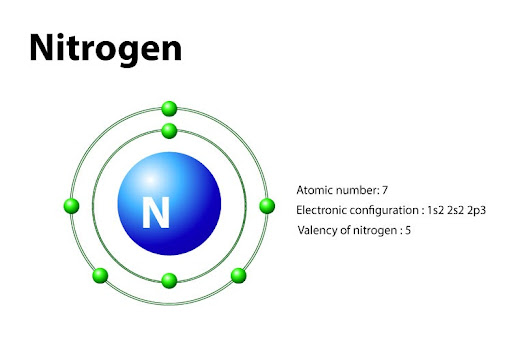# What is the valency of nitrogen?

The valency of an element is a measure of its combining power with other atoms when it forms chemical compounds or molecules. It depends upon the number of valence electrons present in the outermost shell.

Nitrogen:

Nitrogen is an element from Group V of the periodic table. It is a non metal that exists in atmosphere in it's Diatomic form i.e. Nitrogen gas (N2).

The atomic number of nitrogen is 7 and it's

Electronic configuration is 1s2 2s2 2p3.

From which we can conclude that the outermost shell is 2 and the total number of valence electrons is 5; 2 in s orbital and 3 in p orbital.

Thus, the valency of nitrogen is 5. But it mostly has a valency of 3 as it only requires 3 more electrons to complete its octet (octet rule) and form a stable configuration when valence electrons from p orbitals participate in chemical reactions. The s orbital electrons mostly act as a lone pair.

E.g In Ammonia only 3 hydrogens bond with Nitrogen and the s orbital electrons act as lone pairs. So the valency for NH3 is 3 whereas in N2O5 the valency is 5.